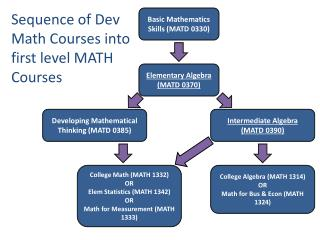Download PresentationBasic Mathematics Skills (MATD 0330)

# Basic Mathematics Skills (MATD 0330)

Download Presentation## Basic Mathematics Skills (MATD 0330)

- - - - - - - - - - - - - - - - - - - - - - - - - - - E N D - - - - - - - - - - - - - - - - - - - - - - - - - - -
##### Presentation Transcript

1. Sequence of Dev Math Courses into first level MATH Courses Basic Mathematics Skills (MATD 0330) Elementary Algebra (MATD 0370) Developing Mathematical Thinking (MATD 0385) Intermediate Algebra (MATD 0390) College Math (MATH 1332) OR Elem Statistics (MATH 1342) OR Math for Measurement (MATH 1333) College Algebra (MATH 1314) OR Math for Bus & Econ (MATH 1324)

2. What Is MATD 0385?

3. Successful Completion Rates in all MATD Courses

4. Successful grade (A, B, C) in MATH 1332 By Gender By Race/Ethnicity